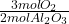## During a laboratory experiment, 36.12 grams of Aboz was formed when O2 reacted with aluminum metal at 280.0 K and 1.4 atm. What was the volu

Question

During a laboratory experiment, 36.12 grams of Aboz was formed when O2 reacted with aluminum metal at 280.0 K and 1.4 atm. What was the volume of O2
used during the experiment?
30 2+ 4A1 — 2AI2O3
8.70 liters
9.22 liters
10.13 liters
12.81 liters

in progress 0
6 months 2021-08-04T08:59:17+00:00 1 Answers 6 views 0

8.70 liters

Explanation:

• 3O₂+ 4Al → 2AI₂O₃

First we convert 36.12 g of AI₂O₃ into moles, using its molar mass:

• 36.12 g ÷ 101.96 g/mol = 0.354 mol AI₂O₃

Then we convert AI₂O₃ moles into O₂ moles, using the stoichiometric coefficients of the reaction:

• 0.354 mol AI₂O₃ *= 0.531 mol O₂

We can now use the PV=nRT equation to calculate the volume, V:

• 1.4 atm * V = 0.531 mol * 0.082 atm·L·mol⁻¹·K⁻¹ * 280.0 K
• V = 8.708 L